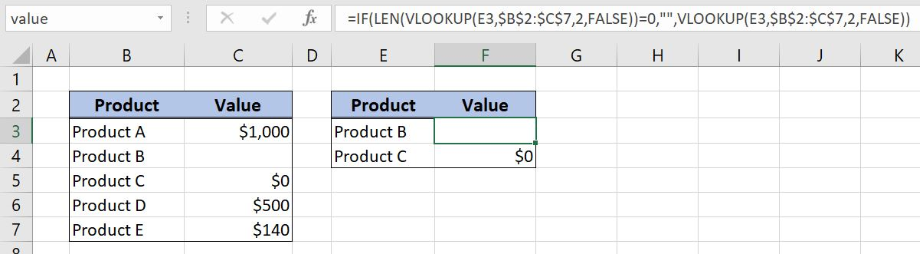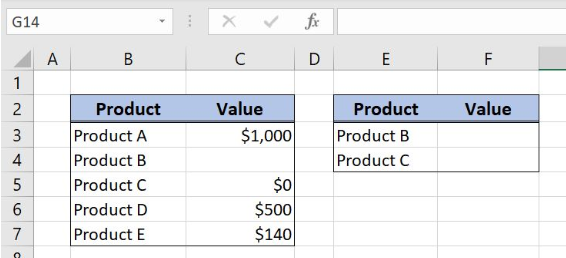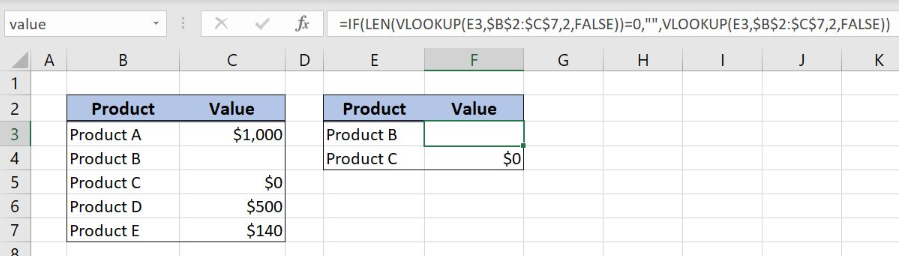Get instant live expert help with Excel or Google Sheets“My Excelchat expert helped me in less than 20 minutes, saving me what would have been 5 hours of work!”

#### Post your problem and you’ll get expert help in seconds.

Your message must be at least 40 characters
Our professional experts are available now. Your privacy is guaranteed.

# Solutions When Your Excel Lookup Returns 0

Excel VLOOKUP retrieves 0 value for the empty cells in the lookup array. To get an empty string as a lookup result we should use several formulas IF, LEN and VLOOKUP. This step by step tutorial will assist all levels of Excel users to get an empty string as a lookup result if the value in the searching column is an empty cell.Figure 1. Excel lookup returns 0 for blank cells

## Syntax of the IF Formula

`=IF(logical_test, value_if_true, value_if_false)`

The parameters of the IF function are:

• logical_test – a logical expression that we want to check
• value_if_true – a value which the function returns if a logical_test is TRUE
• value_if_false – a value which the function returns if a logical_test is FALSE.

## Syntax of the VLOOKUP formula

`=VLOOKUP(lookup_value, table_array, col_index_num, range_lookup)`

The parameters of the VLOOKUP function are:

• lookup_value – a value that we want to find in a table_array
• table_array – a range in which we want to lookup
• col_index_num – a column number in table_array from which we would like to get a value
• range_lookup – default value is FALSE. This means that we want to find an exact match for a lookup value.

## Syntax of the LEN Formula

`=LEN(text)`

The parameter of the LEN function is:

• text – a text for which we want to count the number of characters.

## Setting up Our Data When VLOOKUP Returns 0 for Empty Cells

Our first table consists of 2 columns: “Product” (column B) and “Value” (column C). The second one has the same data structure  “Product” (column E) and “Value” (column F). The idea is to get the value from the first table based on the products from the second table and to place the result in the column Value. If the value in Column C is an empty cell it should be presented as a blank cell into the column F.Figure 2. Table structure for the example

## Convert 0 to Blank Cell when Excel Lookup Returns 0 for Empty Cell

We want to get the value from the first table based on the product criteria from the column E. If the value from column C is an empty cell the result should be also an empty string.

The formula looks like:

`=IF(LEN(VLOOKUP(E3,\$B\$2:\$C\$7,2,FALSE))=0,"",VLOOKUP(E3,\$B\$2:\$C\$7,2,FALSE))`

The logical_test in the IF function is a formula LEN(VLOOKUP(E3,\$B\$2:\$C\$7,2,FALSE))=0 while the value_if_true is an empty string and value_if _false is a formula `VLOOKUP(E4,\$B\$2:\$C\$7,2,FALSE)`. In the LEN function, the parameter text is the formula `VLOOKUP(E3,\$B\$2:\$C\$7,2,FALSE)`.

The lookup_value is the cell E3 while the table_array is the cell range B2:C7. The parameter col_index_num is the number 2 while the range_lookup is FALSE as we want the exact match.

To apply the formula we need to follow these steps:

• Select cell F3 and click on it
• Insert the formula: `=IF(LEN(VLOOKUP(E3,\$B\$2:\$C\$7,2,FALSE))=0,"",VLOOKUP(E3,\$B\$2:\$C\$7,2,FALSE))`
• Press enter
• Drag the formula down to the other cells in the column by clicking and dragging the little “+” icon at the bottom-right of the cell.Figure 3. Solution to get the blank cell result when the value is an empty cell

VLOOKUP function retrieves a 0 value when the value in column C is an empty cell. To convert 0 to an empty string we can use LEN and IF functions. LEN function has the result 0 for the blank cells.

With IF function we are checking if the LEN function result is 0 and if the condition is met, lookup result is an empty string. For non-blank cells, lookup result will be a matchable product value from the column C. Our result is an empty string because Product B value is an empty cell.

Most of the time, the problem you will need to solve will be more complex than a simple application of a formula or function. If you want to save hours of research and frustration, try our live Excelchat service! Our Excel Experts are available 24/7 to answer any Excel question you may have. We guarantee a connection within 30 seconds and a customized solution within 20 minutes.

Solution examplesI filtered data in column A (it is labeled ID #) of a data set. On my next worksheet, column A is also the ID #, but it is a different data set. I want to filter the ID #'s the same for the two sheets, but how do I do that being that I am working with two different sets of data?
Solved by V. H. in 22 minsCould you help me by explaining the IF function?
Solved by G. L. in 24 minsVLOOKUP help. Trying to input data from database into new table
Solved by S. Q. in 40 minsI have a question about Conditional Formatting. =IF('Sheet1'!A1="X",IF('Sheet1'!B1="Y", TRUE, FALSE),FALSE) the set the cell background to red. I would like to make it so that if Sheet1'!B1="Z" I set the back to blue. How can I achieve this.
Solved by B. F. in 39 minsI need to find out if the 2018 yearly sales goals were met if the yearly sales were \$25,000 or more using an IF logical function and to set the formula to return a value of YES if met, and NO if not
Solved by A. A. in 18 mins